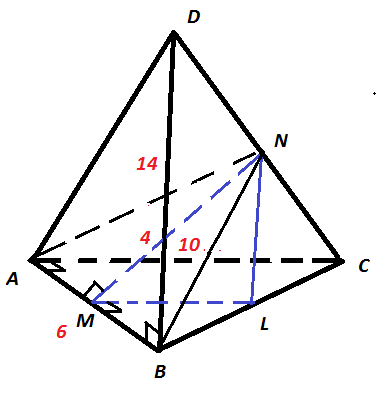# Alright Tetrahedron

Geometry Level 5

In a tetrahedron $ABCD,$ the lengths of $AB,$ $AC,$ and $BD$ are $6,$ $10,$ and $14$ respectively. The distance between the midpoints $M$ of $AB$ and $N$ of $CD$ is $4.$ The line $AB$ is perpendicular to $AC,$ $BD,$ and $MN.$ The volume of $ABCD$ can be written as $a\sqrt{b},$ where $a$ and $b$ are positive integers, and $b$ is not divisible by the square of a prime number. What is the value of $a+b$?×